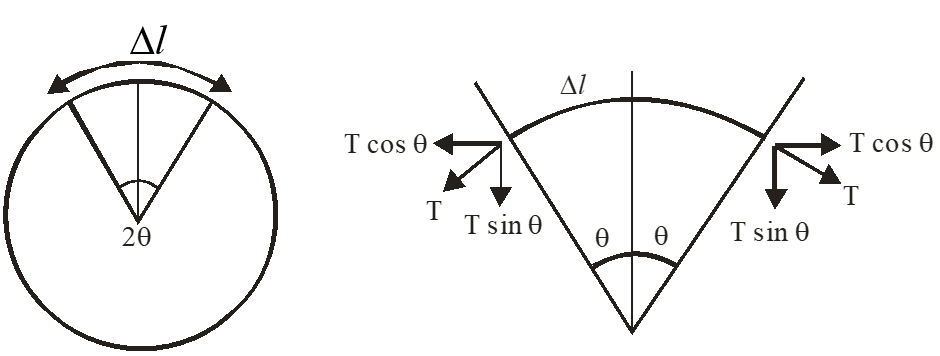Potential energy
Question

# A ring of linear mass density $2\mathrm{\lambda }$  and radius R is rotated about its axis passing through its centre and perpendicular to plane of ring with constant angular speed ‘$\mathrm{\omega }$ ’. The tension in the ring is

Easy
Solution

##Consider an element of length  $\left(\mathrm{\Delta l}\right).$$2\mathrm{Tsin\theta }=\left(2\mathrm{\lambda }\right)\left(\mathrm{\Delta l}\right)\cdot {\mathrm{\omega }}^{2}\mathrm{R}$$2\mathrm{T}\cdot \frac{\left(\mathrm{\Delta l}\right)}{2\mathrm{R}}=\left(2\mathrm{\lambda }\right)\left(\mathrm{\Delta l}\right)\left({\mathrm{\omega }}^{2}\right)\left(\mathrm{R}\right)$$\mathrm{T}=\left(2{\mathrm{\lambda \omega }}^{2}{\mathrm{R}}^{2}\right)$

Get Instant Solutions